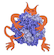IMP  2.3.1 The Integrative Modeling Platform
IMP.pmi.macros.AnalysisReplicaExchange0 Class Reference

A macro for running all the basic operations of analysis. More...

Inherits object.

## Detailed Description

A macro for running all the basic operations of analysis.

Includes clustering, precision analysis, and making ensemble density maps. A number of plots are also supported.

Parameters
 model The IMP model stat_file_name_suffix merge_directories The directories containing output files best_pdb_name_suffix do_clean_first do_create_directories global_output_directory Where everything is replica_stat_file_suffix global_analysis_result_directory
Note
This class is only available in Python.

Definition at line 969 of file macros.py.

## Public Member Functions

def clustering

def get_modeling_trajectory
Get a trajectory of the modeling run, for generating demonstrative movies. More...

## Member Function Documentation

 def IMP.pmi.macros.AnalysisReplicaExchange0.clustering ( self, score_key = 'SimplifiedModel_Total_Score_None', rmf_file_key = 'rmf_file', rmf_file_frame_key = 'rmf_frame_index', prefiltervalue = None, feature_keys = [], outputdir = './', alignment_components = None, number_of_best_scoring_models = 10, rmsd_calculation_components = None, distance_matrix_file = None, load_distance_matrix_file = False, skip_clustering = False, number_of_clusters = 1, display_plot = False, exit_after_display = True, get_every = 1, first_and_last_frames = None, density_custom_ranges = None, write_pdb_with_centered_coordinates = False, voxel_size = 5.0 )

Parameters
 score_key The score for ranking models rmf_file_key Key pointing to RMF filename rmf_file_frame_key Key pointing to RMF frame number prefiltervalue Only include frames where the score key is below this value feature_keys Keywords for which you want to calculate average, medians, etc, outputdir The local output directory used in the run alignment_components List of tuples for aligning the structures e.g. ["Rpb1", (20,100,"Rpb2"), .....] number_of_best_scoring_models Num models to keep per run rmsd_calculation_components List of tuples for calculating RMSD e.g. ["Rpb1", (20,100,"Rpb2"), .....] distance_matrix_file Where to store/read the distance matrix load_distance_matrix_file Try to load the distance matrix file skip_clustering Just extract the best scoring models and save the pdbs number_of_clusters Number of k-means clusters display_plot Display the distance matrix exit_after_display Exit after displaying distance matrix get_every Extract every nth frame first_and_last_frames A tuple with the first and last frames to be analyzed. Values are percentages! Default: get all frames density_custom_ranges List of tuples or strings for density calculation e.g. ["Rpb1", (20,100,"Rpb2"), .....] write_pdb_with_centered_coordinates voxel_size Used for the density output

Definition at line 1067 of file macros.py.

 def IMP.pmi.macros.AnalysisReplicaExchange0.get_modeling_trajectory ( self, score_key = 'SimplifiedModel_Total_Score_None', rmf_file_key = 'rmf_file', rmf_file_frame_key = 'rmf_frame_index', outputdir = './', get_every = 1, nframes_trajectory = 10000 )

Get a trajectory of the modeling run, for generating demonstrative movies.

Parameters
 score_key The score for ranking models rmf_file_key Key pointing to RMF filename rmf_file_frame_key Key pointing to RMF frame number outputdir The local output directory used in the run get_every Extract every nth frame nframes_trajectory Total number of frames of the trajectory

Definition at line 1014 of file macros.py.

The documentation for this class was generated from the following file: